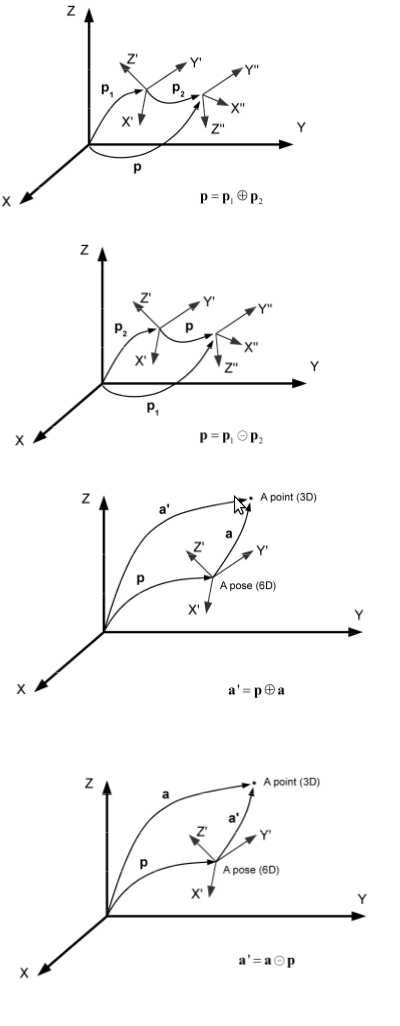[Documentation] [TitleIndex] [WordIndex]

Show EOL distros:

mrpt_common: mrpt_bridge | mrpt_localization | mrpt_map | mrpt_msgs | mrpt_rawlog

# Package Summary

The pose_cov_ops package

mrpt_common: mrpt_bridge | mrpt_libs | mrpt_msgs | pose_cov_ops | rawlog_play

# Package Summary

The pose_cov_ops package

# Package Summary

C++ library for SE(2/3) pose and 2D/3D point composition operations with uncertainty

# Package Summary

Library with C++ functions for SE(2/3) pose and 2D/3D point composition operations with uncertainty

# Package Summary

C++ library for SE(2/3) pose and 2D/3D point composition operations with uncertainty

# Package Summary

C++ library for SE(2/3) pose and 2D/3D point composition operations with uncertainty

## Provided functionality

See the C++ API documentation for the namespace pose_cov_ops.

This module provides implementations for the four main operations between poses (p,p1,p2) and points (a), which are the following (using the "o plus" and "o minus" notation):

1. p1p2 : Composition of two poses p1 and p2: the resulting pose is p2 "as if" p1 was the new origin of coordinates for p2.

2. p2p1 : Inverse composition of two poses p1 and p2: the resulting pose is p2 "as seen from" p1.

3. pa : Composition of a pose p and a point a: the resulting point is a "as if" p was the new origin of coordinates of a.

4. ap : Inverse composition of a pose p and a point a: the resulting point is a "as seen from" p.

Is better to illustrate these operations graphically:## Examples of usage

```   1 #include <mrpt_cov_ops/mrpt_cov_ops.h>
2
3 ...
4 geometry_msgs::PoseWithCovariance p1, p2, p;
5
6 // p = p1 (+) p2
7 // Pose composition, including uncertainty in both p1 and p2:
8 pose_cov_ops::compose(p1,p2,  p);
9
10 ...
11 geometry_msgs::Pose p1;
12 geometry_msgs::PoseWithCovariance p2, p;
13
14 // p = p1 (+) p2
15 // Pose composition, including uncertainty only in p2 (and in the output, obviously!):
16 pose_cov_ops::compose(p1,p2,  p);
```

Further references on the geometry and the maths behind these functions:

• A tutorial on SE(3) transformation parameterizations and on-manifold optimization, J.L. Blanco, Technical report, 2010 PDF.

2019-08-17 13:03